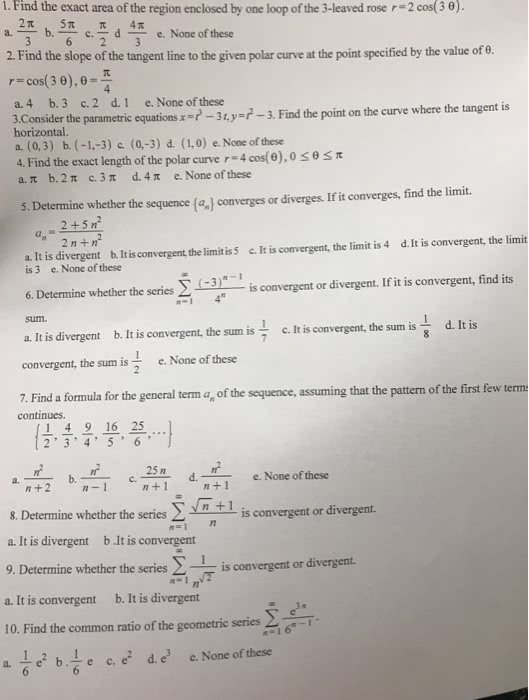1
0
watching
60
views13 Nov 2019

#I. Find the exact area of the region enclosed by one loop of the 3-leaved rose r-2 cos( 3 Î¸). 2, 5Ïã_d 4Ï e. None ofthese 6 2 3 a. b. 2. Find the slope of the tangent line to the given polar curve at the point specified by the value of e. r-cos(30),8-í a. 4 b.3 c.2 d.1 e. None of these 3,Consider the parametric equations-3y-3. Find the point on the curve where the tangent is horizontal a. (0,3) b. (-1.-3) c (0-3) d. (1.0) e. None of these 4, Find the exact length of the polar curve r-4 cos(e),0 a.tb. 2 Ï c, 3 Ï d, 4 Ï e, None of these 5. Determine whether the sequence(%) converges or diverges. If it converges, find the limit. 2+5n a. It is divergent b. Itis convergent, the limitis 5 c. It is convergent, the limit is 4 d.It is convergent, the limit is 3 e. None of these 6 Determine whether the series â½ 3)--1 is convergent or divergent.Ifit is convergent, find its 1 4 sum. a. It is divergent b. It is convergent, the sum is c. It is convergent, the sum is d. It is convergent, the sum is e, None ofthese a formula for the general term a, of the sequence, assuming that the pattern of the first few terms continues. 1 4 9 16 25 2'3'4' 5 6' a. n+1 eNone of these 8. Determine whether the series Î£ a. It is divergent b.It is convergent 9. Determine whether the a. It is convergent b. It is divergent 10. Find the common ratio of the geometric series-T is convergent or divergent. n n=1 series is convergent or divergent. e2b.Åe c.e2 d.e3 e.None ofthese a.